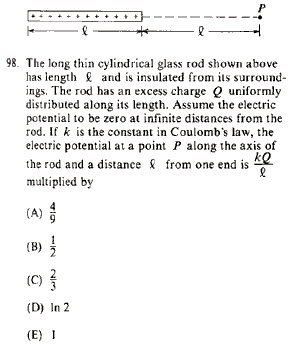GR 8677927796770177 | # Login | Register

GR8677 #98
Problem
 GREPhysics.NET Official Solution Alternate SolutionsElectromagnetism}Potential

Recall that , where is your usual linear charge density.Alternate Solutions
 There are no Alternate Solutions for this problem. Be the first to post one!
casseverhart13
2019-08-09 06:13:52
This problem is so difficult! tree serviceyis5125
2015-09-04 09:22:09
I tried to solve this problem in an alternative way, which is less simple and convenient than the official solution on the site, but unfortunately failed to get the correct answer. I can\'t find my mistake and I am quite baffled. I shall deeply appreciate if someone would like to offer me help soon!\r\n\r\nMy solution is to integrate the intensity of electric field E at the point P first, and then integrate the electric potential at the same point. \r\n\r\nAssume the linear electric density ,\r\nQ=L, dQ=dx,\r\n\r\ndE= =dx, \r\n\r\nE=dx,\r\n\r\nApplying the substitution method, set u=x+l, du=dx, so\r\nE=k=k[]=.\r\n\r\nHence, V===[]=.\r\n\r\nSo my answer is the choice B. \r\n\r\nCan someone tell me where I did wrong?yis5125
2015-09-04 08:42:18
I tried to solve this problem in an alternative way, which is less simple and convenient than the official solution on the site, but unfortunately failed to get the correct answer. I can\'t find my mistake and I am quite baffled. I shall deeply appreciate if someone would like to offer me help soon!\r\n\r\nMy solution is to integrate the intensity of electric field E at the point P first, and then integrate the electric potential at the same point. \r\n\r\nAssume the linear electric density ,\r\nQ=L, dQ=dx,\r\ndE=\r\n =dx, \r\nE= dx,\r\nApplying the substitution method, set u=x+l, du=dx, so\r\nE=k=k[]=.\r\n\r\nHence V=\r\n =.\r\nFinally, there comes out infinity of the electric potential. \r\n\r\nCan someone tell me where I did wrong? \r\nLawliet_Black
2013-08-24 03:44:40
If I'm thinking of this correctly, the answer should be greater than 1/2 (it would be as if all of the charges were at 2l) and less than 1 (as if all the charges were at l), so you can eliminate all but C and D.anum
2010-11-11 12:21:44
i don't get it why not b?
 anum2010-11-11 12:23:10 i mean e. why involve integration.
 flyboy6212010-11-15 20:40:24 You have to integrate because each dq contributes differently to the potential according to its distance from P. Integrating over from to gives (D).rohit
2008-11-07 07:52:44
firstly , the limits are from 0 to l ( over the charge distribution )
second, the the integrand is dq/(x+l)

 wittensdog2009-07-28 10:51:15 Either method should be equivalent. In the official solution, x represents the distance from the point P to a point on the rod, since the potential goes like 1/x from a point charge. The closest point is a distance l away, and the furthest is a distance 2l away. So integrating over the rod entails integrating from l to 2l, since the integration variable is x. Your method merely represents a substitution x -> x + l, with the limits accordingly shifted. You use x instead to represent not the distance from the point P to a point on the rod, but as a variable that ranges over the length of the rod, and thus indeed, x+l becomes the denominator in the potential, since now this is the distance to point P. Both methods yield the correct answer. Depending on the order of integration you could have a sign difference, but clearly that doesn't matter for finding the right answer in this problem. Actually, in order to evaluate the integral you propose, you would likely make a substitution of the form u = x + l, so that you would have an integral of the form du / u, and then shift the limits of integration so that they were in terms of u. This would restore the original integral in the official solution.
 jmracek2009-10-16 19:17:56 i think rohit's approach is more intuitive
 spacemanERAU2009-10-21 18:10:13 it is more intuitive but requires a little more worka19grey2
2008-11-04 16:41:12
To clarify, the correct answer is D.Andresito
2006-03-21 00:48:48
V = k * integral (dq / x) , in the first expression.This problem is so difficult! tree serviceLaTeX syntax supported through dollar sign wrappers $, ex.,$\alpha^2_0$produces . type this... to get...$\int_0^\infty\partial\Rightarrow\ddot{x},\dot{x}\sqrt{z}\langle my \rangle\left( abacadabra \right)_{me}\vec{E}\frac{a}{b}\$